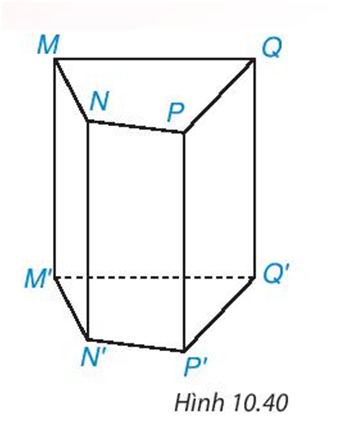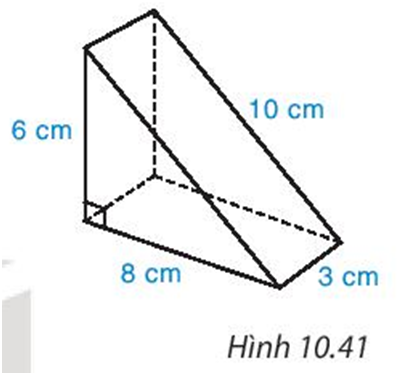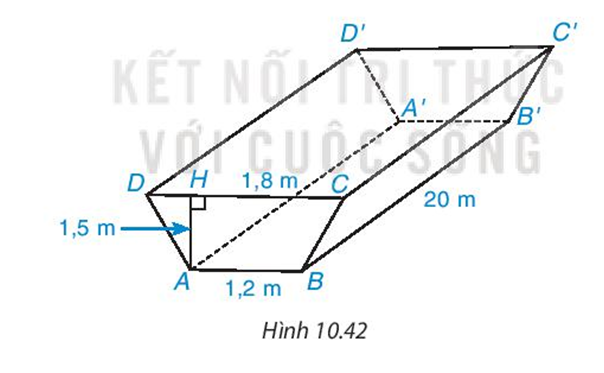## Solve the exercises Exercise page 101 (Chapter 10 Math 7 Connect) – Math Book

Solve the Exercise Exercise page 101 (Chapter 10 Math 7 Connect)

———

10.17. Write the names of the vertices, sides, sides, and bases of the rectangular prism in Figure 10.40 .Solution guide:

+ 8 vertices: M, N, P, Q, M’, N’, P’, Q’.

+ 12 edges: MN, MQ, NP, PQ, M’N’, M’Q’, N’P’, P’Q’, MM’, NN’, PP’, QQ’.

+ 4 sides: MNN’M’, NPP’N’, PQQ’P’, MQQ’M’.

+ 2 bottom faces: MNPQ, M’NP’Q’.

10.18. A cake has the shape of a triangular prism, the size is as shown in figure 10.41

a) Calculate the volume of the cake.

b) If a box has to be made to fit this cake, what is the area of ​​material needed (regardless of the gluing edge).Solution guide:

a) The volume of the cake is the volume of a prism whose base is a right triangle with sides equal to 6 cm and 8 cm.

The volume of the prism is:

$\frac{1}{2}$. 6. 8. 3= 72 (cm3)

b) The area of ​​material to be used is the area around the prism and the area of ​​the two bases.

Using the Pythagorean theorem, the remaining side of the triangle at the base is: $\sqrt{6^{2}+8^{2}}=10$.

The amount of material needed is:

3. (6 + 8 + 10) + $\frac{1}{2}$. 6. 8 = 96 (cm2).

10.19. People dig a ditch in the shape of a prism standing quadrilateral as shown in Figure 10.42. The ditch is 20 m long, 1.5 m deep, 1.8 m wide on the surface and 1.2 m wide at the bottom. Calculate the volume of soil to be excavatedSolution guide:

The volume of soil to be excavated is the volume of the rectangular prism ABCD. A’B’C’D’

The area of ​​the base ABCD is:

$\frac{1}{2}$.1.5. (1.8 + 1.2) = 2.25 (m2)

Volume of a vertical prism of quadrilateral ABCD. A’B’C’D’ is :

2.25. 20 = 45 (m3).